PROJECTS Arduino

## POV Display Using SPRESENSE

DFRobot Mar 27 2019 495

### Story

#### One AA battery was used for the motor and three AA batteries for the wireless power supply to the rotating part.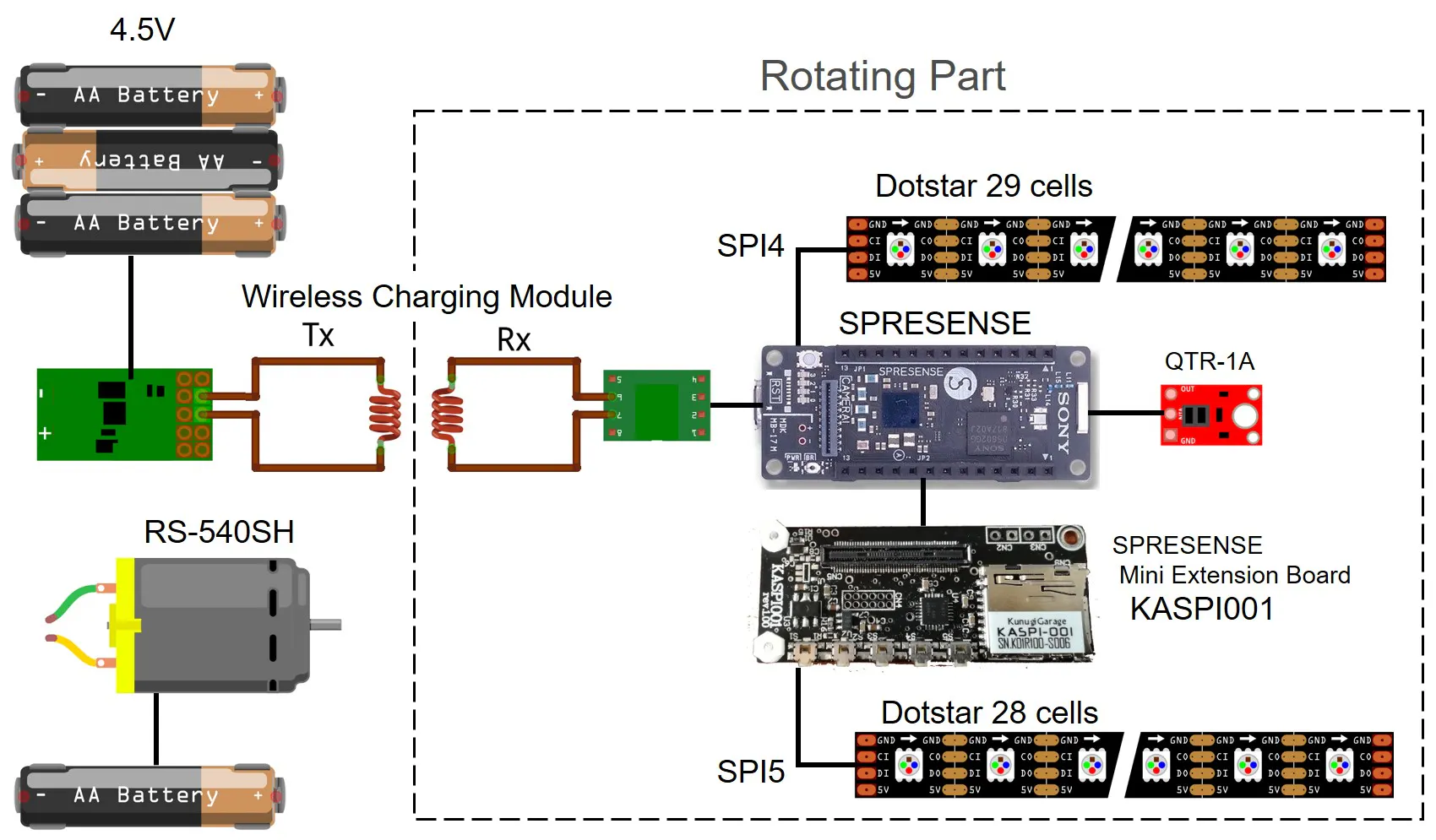#### POV Display DeviceThe motor was fixed with metal fittings and the handle was made with black wood, and the battery socket and power supply on/off slide switch were fixed.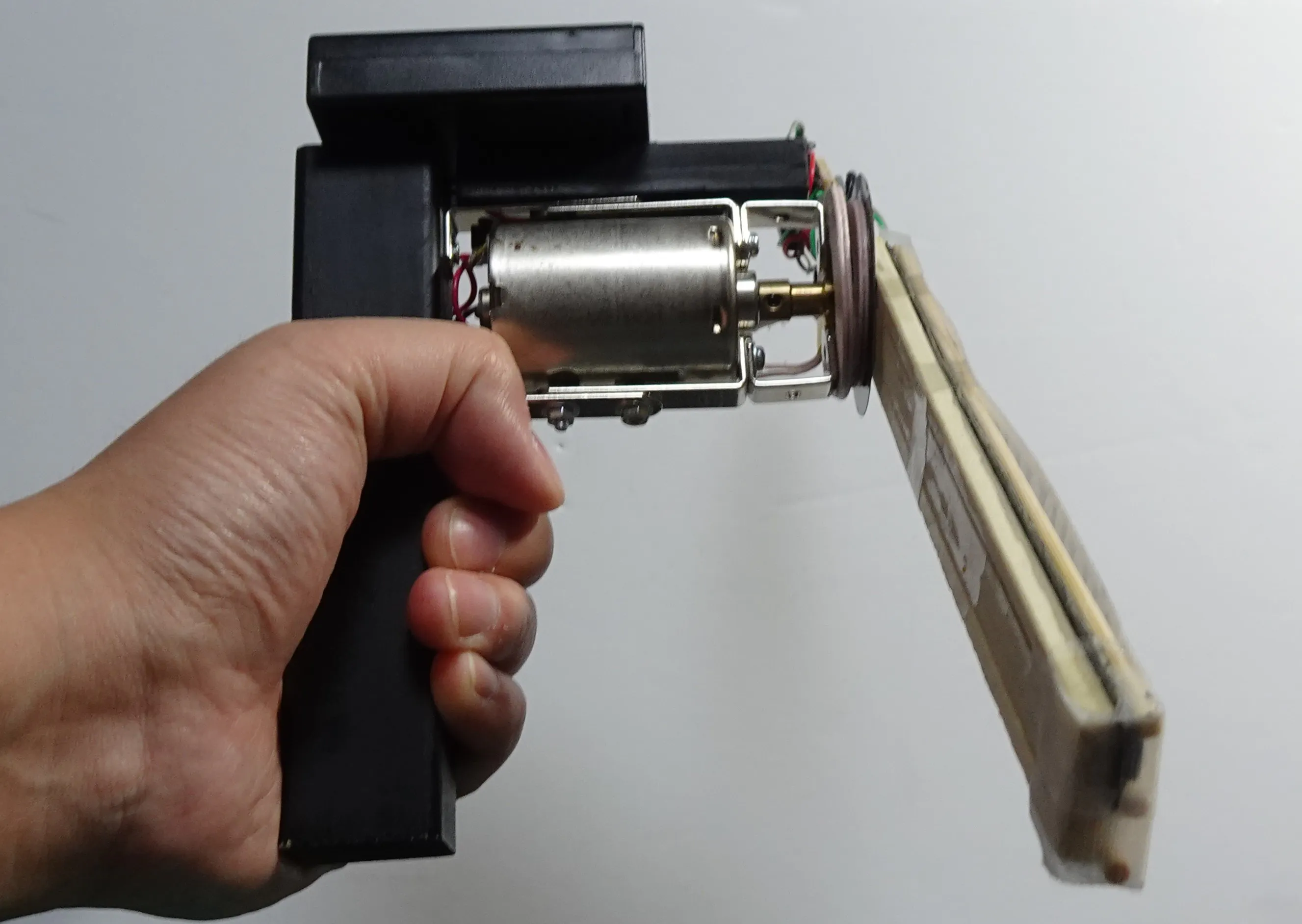#### The rotating part is completely independent as follows.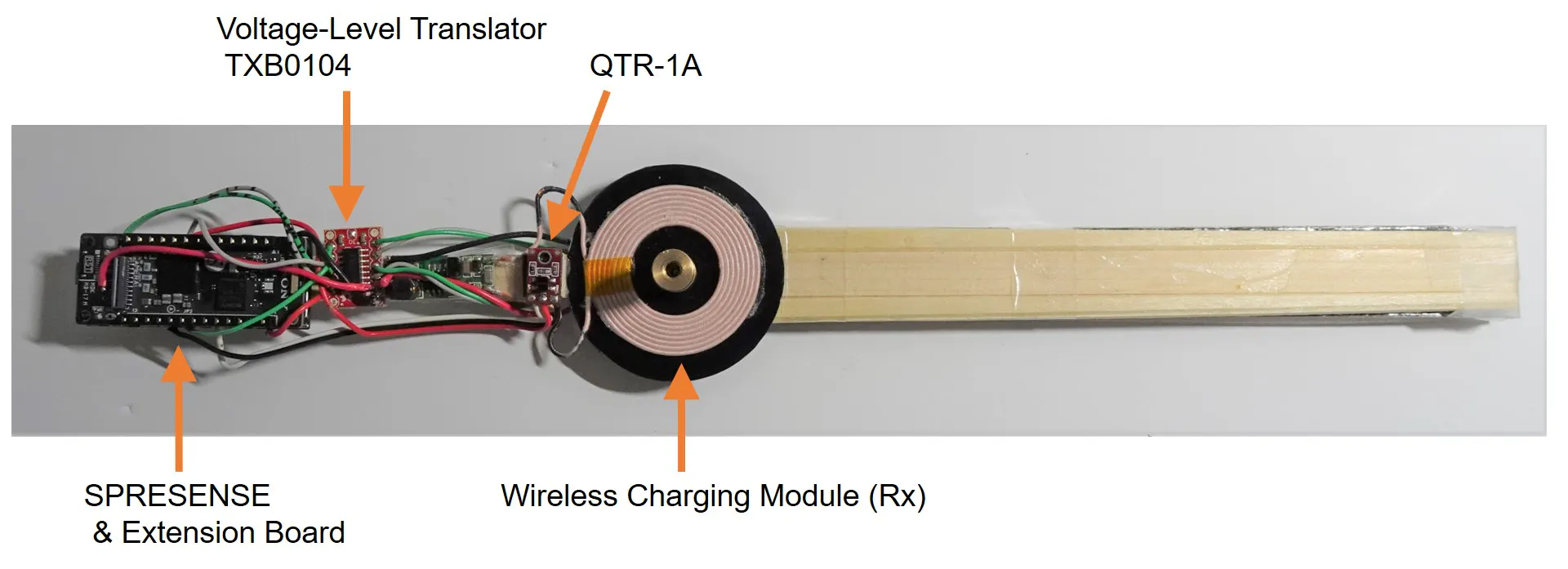#### I used two Dotstar LED tapes. 29 cells LED tape is controlled by SPI4. 28 cells LED tape is controlled by SPI5.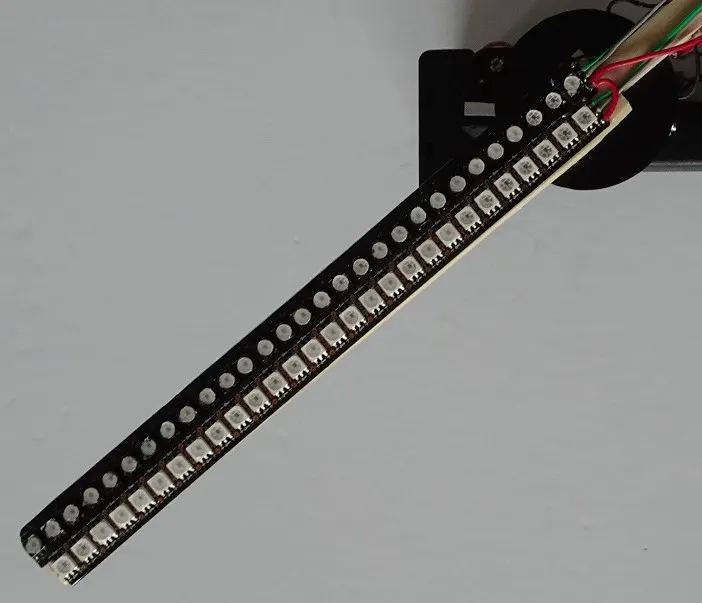#### I spread a milky film for the diffusion of light.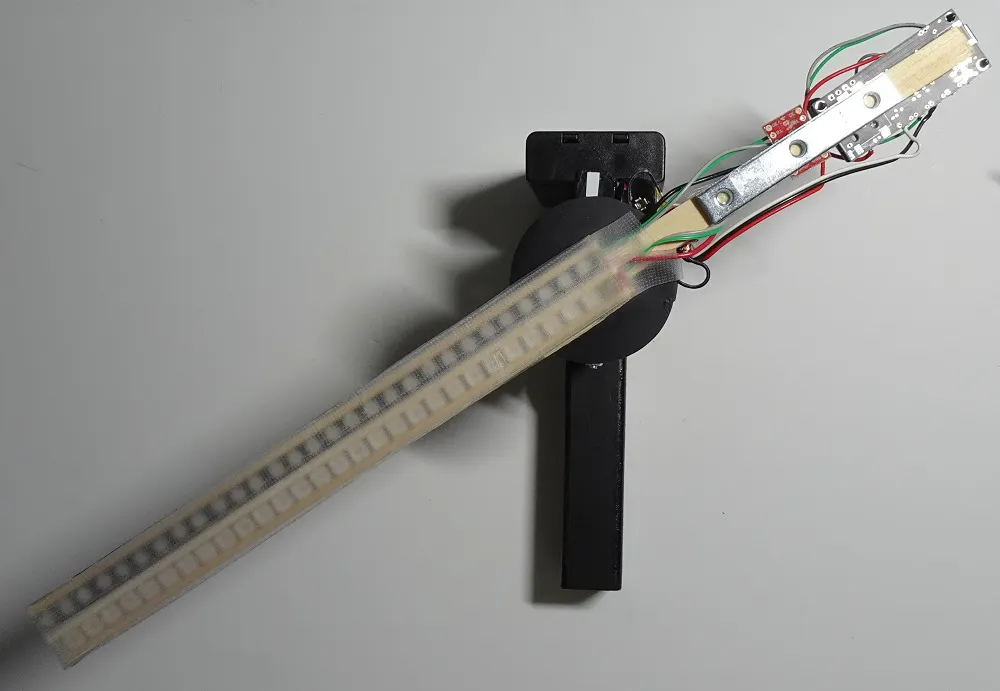#### Voltage-Level Translator TXB0104 is used to convert the logic level of SPRESENSE from 1.8V to 5V.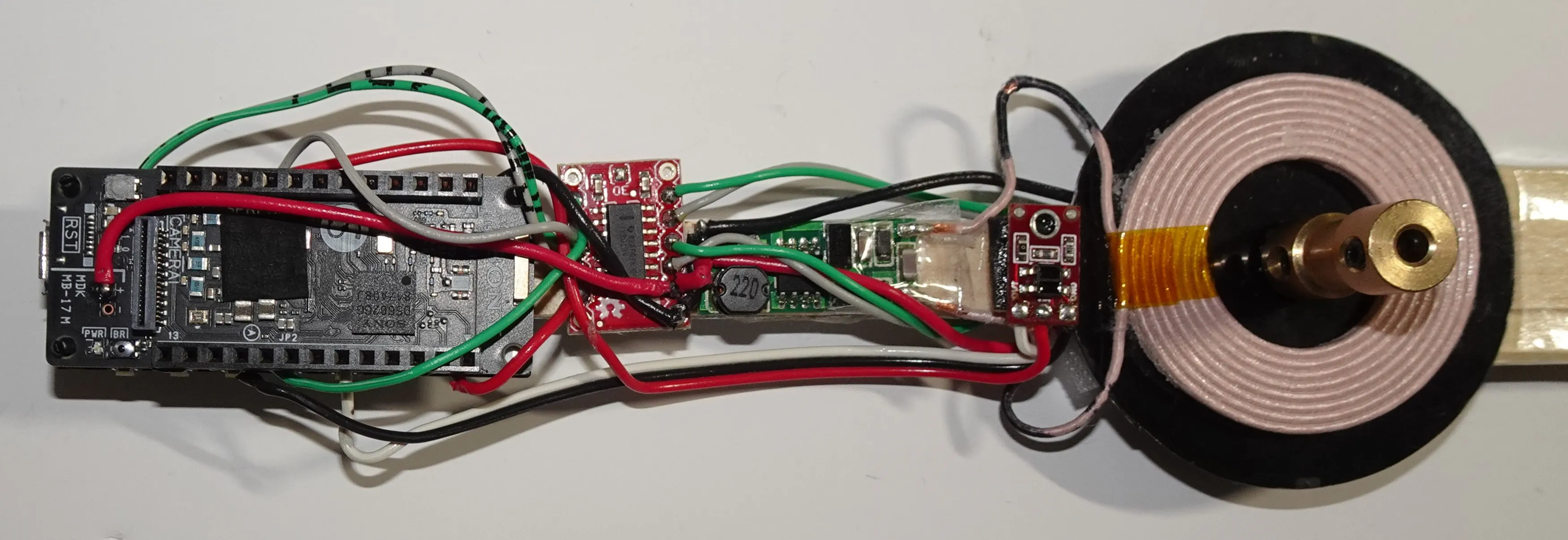#### I used the wireless charge module for power supply to the LED rotating part. It is only placed in the face-through the transmission coil to the rotation axis of the motor without almost processing.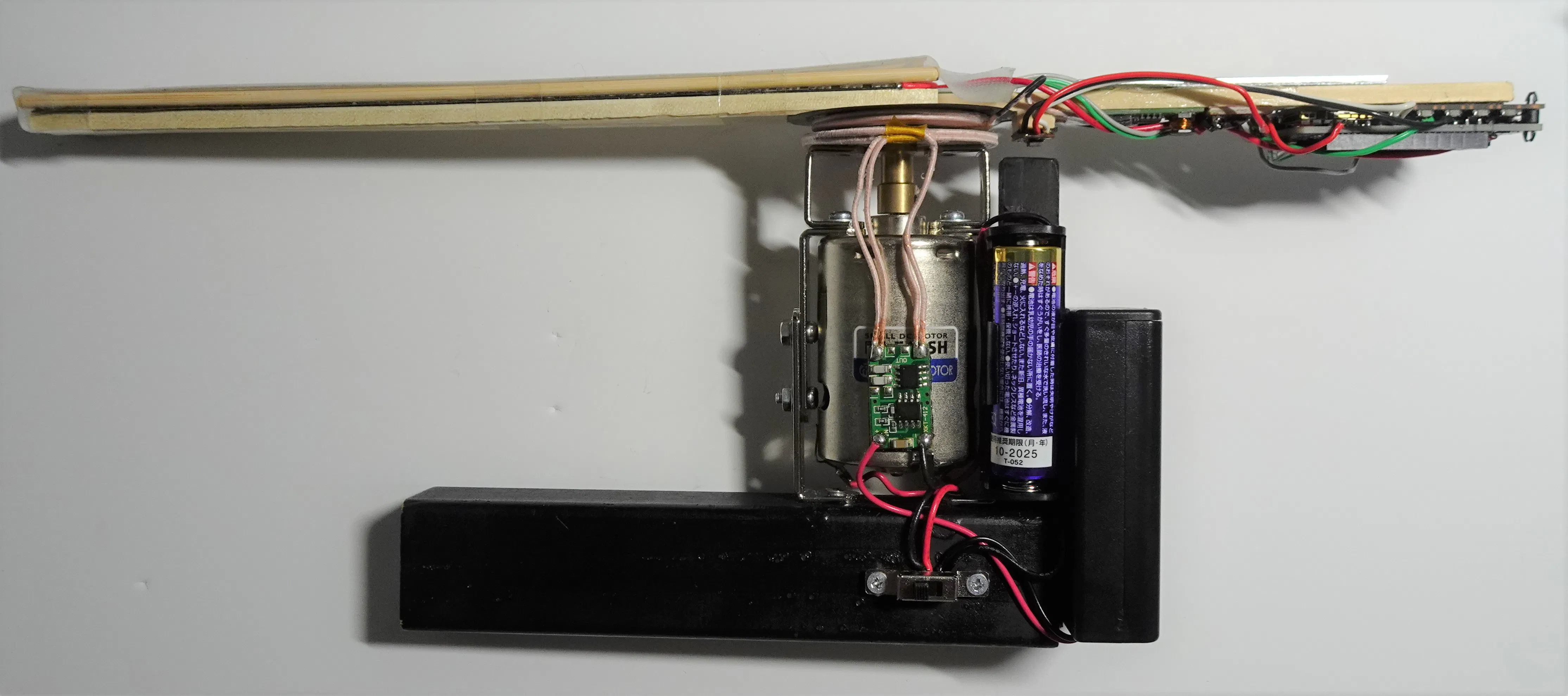### Arduino IDE Code

#### When a reflectance sensor detects a marker, it decreases the output, so it detects it in the attachinterrupt and measures the time it takes to interrupt processing by one lap.It switches the LED blink by dividing the time of one lap by 150. LED display patterns are stored as an array in graphics.h.

```#include <SPI.h>
#include "graphics.h"
#define NUMPIXELS2 29 // Number of LEDs in strip
#define NUMPIXELS 57 // Number of LEDs in 2 strips
#define Frame 16
#define Div 150
#define Fclk 26000000
#define itrPin 19
int numRot = 0;
int numDiv = 0, numDiv2 = 0;
int stateDiv = 0;
unsigned long rotTime, timeOld, timeNow;
void setup() {
Serial.begin(115200);
strip5.begin();
SPI5.beginTransaction(SPISettings(Fclk, MSBFIRST, SPI_MODE0));
strip4.begin();
SPI.beginTransaction(SPISettings(Fclk, MSBFIRST, SPI_MODE0));
strip5.clear();
strip4.clear();
strip5.show();
strip4.show();
delay(500);
attachInterrupt(digitalPinToInterrupt(itrPin), RotCount, FALLING );
}
void loop() {
if(stateDiv == 1 && micros() - timeOld > rotTime / Div * (numDiv)){
stateDiv = 0;
}
if(stateDiv == 0 && micros() - timeOld < rotTime / Div * (numDiv + 1)){
stateDiv = 1;
strip5.clear();
strip4.clear();
for(int i=0;i<NUMPIXELS2; i++){
numDiv2 = numDiv+2;
if(numDiv2 >= Div) numDiv2 -= Div;
strip5.setPixelColor(i, pic[numRot][numDiv2][i*2]);
strip4.setPixelColor(i, pic[numRot][numDiv][i*2+1]);
}
strip5.show();
strip4.show();
numDiv++;
if(numDiv >= Div ) numDiv = 0;
}
}
void RotCount() {
timeNow = micros();
rotTime = timeNow - timeOld;
timeOld = timeNow;
numRot++;
if(numRot >= Frame) numRot = 0;
}```

#### Create POV display data "graphics.h" in Python Code. Display data can be created from GIF or still images.

```# -*- coding: utf-8 -*-
import cv2
import os
import math
from PIL import Image
#Array setting
NUMPIXELS = 57 #Number of LEDs
Div = 150 #Number of divisions per lap
Bright = 30 #LED Brightness
Led0Bright = 3 #Brightness of center LED [%]
#File creation
file = open('graphics.h', 'w')
file.write('#define NUMPIXELS ' + str(NUMPIXELS) + '\n')
file.write('#define Div ' + str(Div) + '\n' + '\n')
#file.write('#define Frame ' + str(Frame) + '\n' + '\n')
file.write('const uint32_t pic [Frame][Div][NUMPIXELS] = {' + '\n')
gif_file_name = "xxx.gif"
gif = cv2.VideoCapture(gif_file_name)
#Image conversion function
def polarConv(pic, i):
h, w, _ = imgOrgin.shape #Get image size
#Image reduction
imgRedu = cv2.resize(imgOrgin,(math.floor((NUMPIXELS * 2 -1)/h *w), NUMPIXELS * 2 -1))
#cv2.imwrite(str(i) + '-resize.jpg',imgRedu)
#Reduced image center coordinates
h2, w2, _ = imgRedu.shape
wC = math.floor(w2 / 2)
hC = math.floor(h2 / 2)
#Polar coordinate conversion image preparation
imgPolar = Image.new('RGB', (NUMPIXELS, Div))
#Polar transformation
file.write('\t{\n')
for j in range(0, Div):
file.write('\t\t{')
for i in range(0, hC+1):
#Get coordinate color
rP = int(imgRedu[hC + math.ceil(i * math.cos(2*math.pi/Div*j)),
wC - math.ceil(i * math.sin(2*math.pi/Div*j)), 2]
* ((100 - Led0Bright) / NUMPIXELS * i + Led0Bright) / 100 * Bright /100)
gP = int(imgRedu[hC + math.ceil(i * math.cos(2*math.pi/Div*j)),
wC - math.ceil(i * math.sin(2*math.pi/Div*j)), 1]
* ((100 - Led0Bright) / NUMPIXELS * i + Led0Bright) / 100 * Bright /100)
bP = int(imgRedu[hC + math.ceil(i * math.cos(2*math.pi/Div*j)),
wC - math.ceil(i * math.sin(2*math.pi/Div*j)), 0]
* ((100 - Led0Bright) / NUMPIXELS * i + Led0Bright) / 100 * Bright /100)
file.write('0x%02X%02X%02X' % (rP,gP,bP))
if i == hC:
file.write('},\n')
else:
file.write(', ')
imgPolar.putpixel((i,j), (rP, gP, bP))
file.write('\t},\n\n')
#Generate directory to save screen capture
dir_name = "screen_caps"
if not os.path.exists(dir_name):
os.mkdir(dir_name)
i = 0
while True:
# Exit when the file can not be read
if not is_success:
break
# Write out to an image file
img_name = str(i) + ".jpg"
img_path = os.path.join(dir_name, img_name)
cv2.imwrite(img_path, frame)
#conversion
polarConv(img_path, i)
i += 1
file.write('};' + '\n' + '\n')
file.close()
#Inserting the number of frames at the beginning of the file
with open('graphics.h') as f: